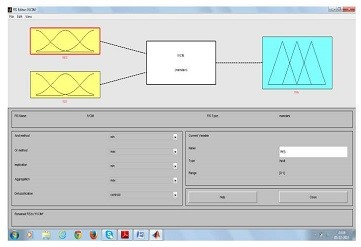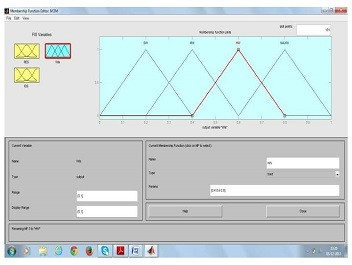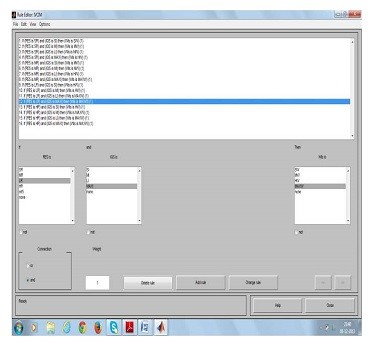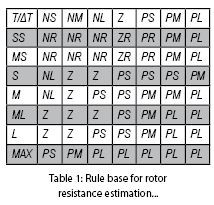Speed Control Of Induction Motor Drive

Recently, attention has been given to the identification of the instantaneous value of the rotor resistance while the drive is in normal operation... - P V Narendra KumarIndirect Field Oriented Controlled (IFOC) induction motor drives are being increasingly used in high-performance drive systems, as induction motors are more reliable because of their construction and less expensive materials used, than any other motors available in the market today. As indirect field orientation utilises an inherent slip relation, it is essentially a feed forward scheme and hence depends greatly on the accuracy of the motor parameters used in the vector controller – particularly to the rotor resistance. It changes widely with the rotor temperature, resulting in various harmful effects – such as over (or under) excitation, the destruction of the decoupled condition of the flux and torque, etc. Recently, attention has been given to the identification of the instantaneous value of the rotor resistance while the drive is in normal operation. So far, several approaches have been presented. A new sliding mode current observer for an induction motor is developed. Sliding mode functions are chosen to determine speed and rotor resistance of an induction motor in which the speed and rotor resistance are assumed to be unknown constant parameters. In, a method using a programmable cascaded low pass filter for the estimation of rotor flux of an induction motor, with a view to estimate the rotor time constant of an indirect field orientation controlled induction motor drive, is investigated. The estimated rotor flux data has also been used for the on-line rotor resistance identification with artificial neural network. Despite all these effects, rotor resistance estimation remains a difficult problem.

Indirect Field-Oriented Induction Motor Drive

The indirect vector control method is essentially same as the direct vector control, except that the unit vector generated in an indirect manner using the measured speed r and slip speed sl. The following dynamic equations are taken into consideration to implement indirect vector control strategy.

θe = ωe dt = (ωr + ωsl) = θr + θsl
Slip frequency can be calculated as
ωsl = Lm Rr iqs
Lr

For constant rotor flux ψr and dψr/dt=0, substituting in equation yields the rotor flux set as ψr = Lm idsFig 1: Block Diagram of Fuzzy Logic Controller…

Design of Fuzzy Logic Controller for Induction Motor Drive

Fig. 1 shows block diagram of speed control system using Fuzzy Logic Controller (FLC). Here, the first input is the Temperature ‘T’ and second is the Change in Temperature ‘ΔT’ at sampling time ‘ts.’ The two input variables ‘T’ and ‘ΔT’ are calculated at every sampling time as functional block diagram of Fuzzy Logic Control. In this block, number of inputs and number of outputs are estimated and any relationship is appeared between inputs and outputs are checked. The crisp values for the inputs and outputs are noted down. The entire information regarding the application to be solved is noted down here. The rotor resistance with respect to temperature & change in temperature are noted down here.

Where ‘wls’ denotes the Slip frequency, r *(ts) is the reference rotor speed, r(ts) is the actual speed, e(ts-1) is the value of error at previous sampling time. The output variable is the rotor resistance. As shown in Fig. 2, the Fuzzy Logic Controller consists of four blocks, Fuzzification, inference mechanism, knowledge base and Defuzzification.Fig 2: Fuzzy Logic Controller Membership Functions…

• Fuzzification Block: In this stage the crisp variables of input are converted into fuzzy variables. The fuzzification maps the error and change in error to linguistic labels of fuzzy sets. Membership function is associated to each label with triangular shape which consists of two inputs and one output. The proposed controller uses following linguistic labels. Each of the inputs and output contain membership function with all these seven linguistics.
• Knowledge Base & Inference Stage: Knowledge base involve defining the rules represented as ‘if-then’ rules statements governing the relationship between input and output variables in terms of membership function. In this stage, the input variables ‘T’and ‘ΔT’ are processed by the inference mechanism that executes 49 rules represented in rule table shown below. Considering the first rule, if temperature is NS and change in temperature is SS, then the output will be NR. Here, Mamdani’s algorithm for inference mechanism used.
Figure 2 shows the configuration of the proposed fuzzy logic rotor resistance estimation. The functions F and F0 are first calculated respectively from the estimated variables ids; iqs; vds; vqs; !e and the reference value. The inputs temperature and change in temperature are the variables, which are used as inputs for the FLC. The internal structure of the fuzzy logic rotor resistance estimation is chosen similar to that of a Fuzzy Logic Controller, which consists of fuzzification, inference engine and defuzzification. For the successful design of FLC’s proper selection of these gains are crucial jobs, which in many cases are done through trial and error to achieve the best possible control performance. Then the crisp variables are converted into fuzzy variables using triangular membership functions as in Figure 3. These input membership functions are used to transfer crisp inputs into fuzzy sets.
The expert’s experience is incorporated into a knowledge base with 49 rules (7×7). This experience is synthesised by the choice of the input-output (I/O) membership functions and the rule base. Then, in the second stage of the FLC, the inference engine, based on the input fuzzy variables, uses appropriate IF-THEN rules in the knowledge base to imply the final output fuzzy sets as shown in the Table 1, where NS, NM, NL, Z, PS, PM, PL correspond to Negative Small, Negative Medium, Negative Large, Zero, Positive Small, Positive Medium, Positive Large respectively.Fig 3: Rule Base Editor…

• Defuzzification: This stage introduces different methods that can be used to produce fuzzy set value for the output fuzzy variable T. Here the centre of gravity or centroids method is used to calculate the final fuzzy value. Defuzzification using COA method – means that crisp output is obtained by using centre of gravity – in which the crisp output variable is taken to be the geometric centre of the output fuzzy variables where it is formed by taking the union of all the contributions of rules with the degree of fulfillment greater than zero. Then the COA expression with discretised universe of discourse can be Te * – obtained by integration which is used to calculate variation of rotor flux, where it is observed that in case of PI controller it will take more time to reach to steady state value. But in case of fuzzy controller it will take less time to reach steady value.
In the defuzzification stage, the implied fuzzy set is transformed to a crisp output by the center of gravity defuzzification technique as given by the formula (19), zi is the numerical output at the ith number of rules and _(zi) corresponds to the value of fuzzy membership function at the ith number of rules. The summation is from one to n, where n is the number of rules that apply for the given fuzzy inputs. This value added to the reference rotor time constant (Tref) gives the estimated time constant (Tr), which is used as an input to the F.O.C. block of Figure 1 to ensure the correct orientation operation of the drive. Without considering the effects derived from the saturation (Lr constant), Rr−est is obtained from the estimated rotor time constant Figure 2. Therefore, this rotor resistance estimation value used in the control model must match its real value in order to maintain a high performance of the induction motor drive as will be shown later. The input/output mapping of the FLC rotor resistance estimation is shown in Figure 4, which is a continuous highly non-linear function. Detailed discussion about FLC construction is referred in.Simulation Results

The configuration of the overall control system is shown in Figure 1. It is essential that the simulation model is designed to approach as close to reality as possible. Therefore, for the simulation of the whole drive system according to Figure 1, a mathematical model has been developed based on the induction motor equations and the equations for estimating the rotor resistance which have been derived in Section III. In addition, a mathematical model for all the remaining drive system units was necessary to complete the simulation model.

In order to analyse the drive system performance for their flux and torque responses, with rotor resistance variation, the above-presented system has been simulated using MATLAB/SIMULINK software.

A squirrel cage induction motor with a rated power of 1.5 kW has been used. A constant reference flux of 0.695 Wb is assumed and the speed was held constant at 1000 rpm.

The rotor resistance was stepped or ramped from 100 to 200% of its rated value, thereby simulating a change in rotor resistance due to a temperature change. The system was first started up to 1000 rpm with a full load of 10 N.m. At 1.5 sec, the rotor resistance was stepped from 100 to 200% of its rated value.

It is observed in this figure that when the estimated rotor resistance deviates from its real value, the field orientation scheme is detuned and the command torque (Te) instead of stabilising at its rated value, it is increased to 17 N.m to compensate the drop in speed which equals approximately 12 rpm.

But in the actual operating conditions, the rate of change of temperature is very slow and so the resistance variation. Accordingly, at 1.5 sec a ramp change of rotor resistance for an uncompensated case is applied linearly from 100% of its rated value to 200% till 4.5 sec, then, this value is maintained for 2.5 sec.